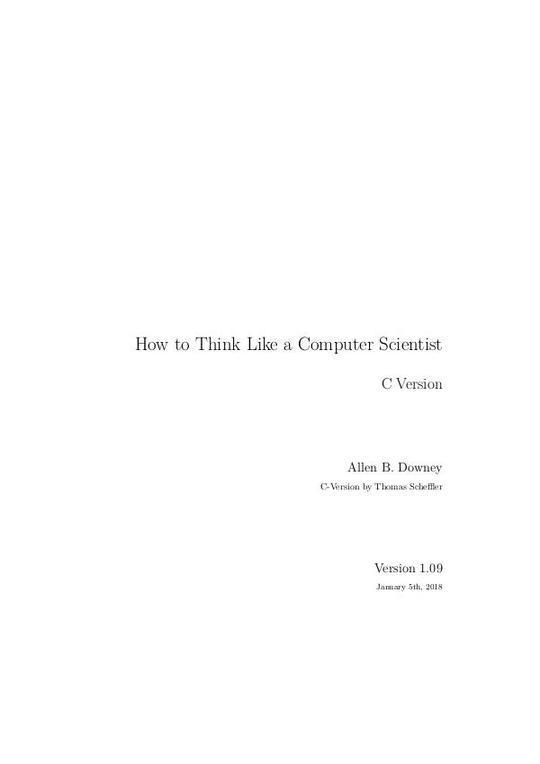Free

# Think-C_v1.09

By Unknown
Free
The publisher has enabled DRM protection, which means that you need to use the BookFusion iOS, Android or Web app to read this eBook. This eBook cannot be used outside of the BookFusion platform.
Book Description
• The way of the program
• What is a programming language?
• What is a program?
• What is debugging?
• Compile-time errors
• Run-time errors
• Logic errors and semantics
• Experimental debugging
• Formal and natural languages
• The first program
• Glossary
• Exercises
• Variables and types
• More output
• Values
• Variables
• Assignment
• Outputting variables
• Keywords
• Operators
• Order of operations
• Operators for characters
• Composition
• Glossary
• Exercises
• Function
• Floating-point
• Constants
• Converting from double to int
• Math functions
• Composition
• Definitions and uses
• Programs with multiple functions
• Parameters and arguments
• Parameters and variables are local
• Functions with multiple parameters
• Functions with results
• Glossary
• Exercises
• Conditionals and recursion
• Conditional execution
• The modulus operator
• Alternative execution
• Chained conditionals
• Nested conditionals
• The return statement
• Recursion
• Infinite recursion
• Stack diagrams for recursive functions
• Glossary
• Exercises
• Fruitful functions
• Return values
• Program development
• Composition
• Boolean values
• Boolean variables
• Logical operators
• Bool functions
• Returning from main()
• Glossary
• Exercises
• Iteration
• Multiple assignment
• Iteration
• The while statement
• Tables
• Two-dimensional tables
• Encapsulation and generalization
• Functions
• More encapsulation
• Local variables
• More generalization
• Glossary
• Exercises
• Arrays
• Increment and decrement operators
• Accessing elements
• Copying arrays
• for loops
• Array length
• Random numbers
• Statistics
• Array of random numbers
• Passing an array to a function
• Counting
• Checking the other values
• A histogram
• A single-pass solution
• Random seeds
• Glossary
• Exercises
• Strings and things
• Containers for strings
• String variables
• Extracting characters from a string
• Length
• Traversal
• Finding a character in a string
• String concatenation
• Assigning new values to string variables
• strings are not comparable
• Character classification
• Getting user input
• Glossary
• Exercises
• Structures
• Compound values
• Point objects
• Accessing member variables
• Operations on structures
• Structures as parameters
• Call by value
• Call by reference
• Rectangles
• Structures as return types
• Passing other types by reference
• Glossary
• Exercises
• Coding Style
• A short guide on style
• Naming conventions and capitalization rules
• Bracing style
• Layout
• ASCII-Table
You May Also Like